# Quasi-derivative

Known as: Quasi-differentiable, Quasiderivative, Quasidifferentiable
In mathematics, the quasi-derivative is one of several generalizations of the derivative of a function between two Banach spaces. The quasi… Expand
Wikipedia

## Papers overview

Semantic Scholar uses AI to extract papers important to this topic.
2015
2015
In the article we use abstract convexity theory in order to unify and generalize many different concepts of nonsmooth analysis… Expand
Is this relevant?
2002
2002
In this paper, we propose a new method for the unconstrained minimization of a function presented as a difference of two convex… Expand
•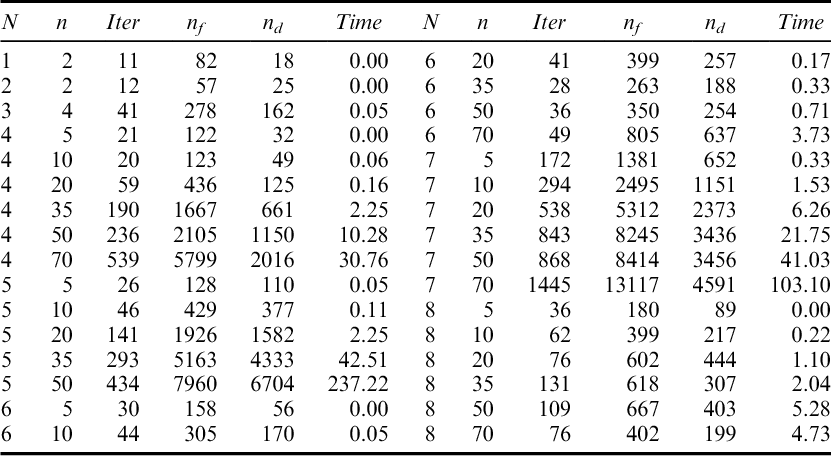Is this relevant?
2000
2000
In the first part of this paper, the Demyanov difference of two sets is considered. An expression for the Demyanov difference of… Expand
Is this relevant?
1994
1994
• Math. Program.
• 1994
A new generalized Farkas theorem of the alternative is presented for systems involving functions which can be expressed as the… Expand
Is this relevant?
1993
1993
• Math. Program.
• 1993
The classical linearization procedure for differentiable nonlinear programming problems can be naturally generalized to the… Expand
Is this relevant?
1992
1992
SummaryIn this paper several new types of variational principles are derived by using the new mathematical notion of… Expand
•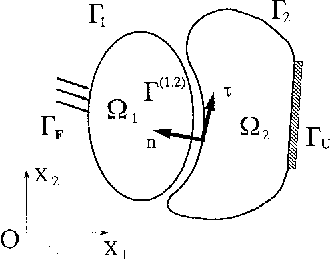•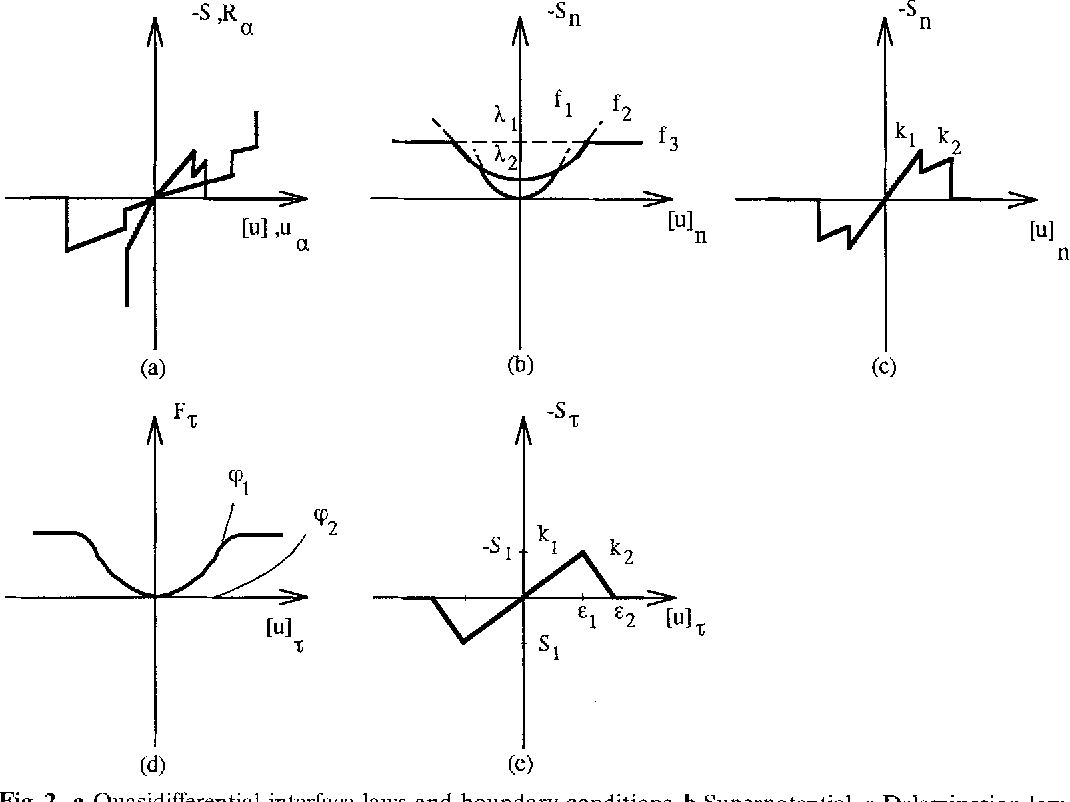•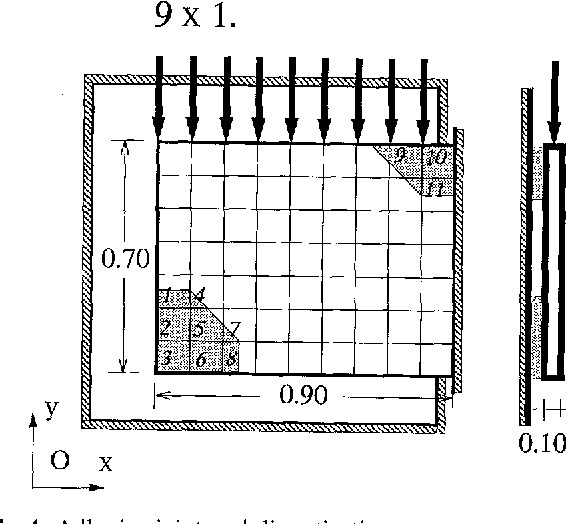•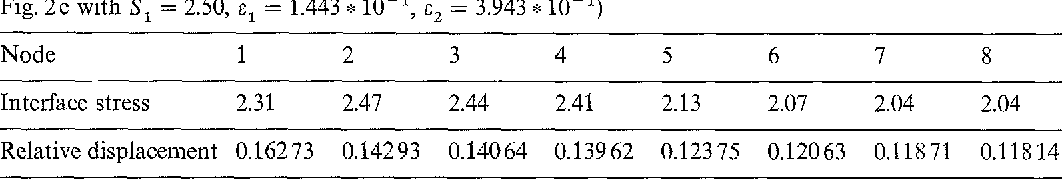•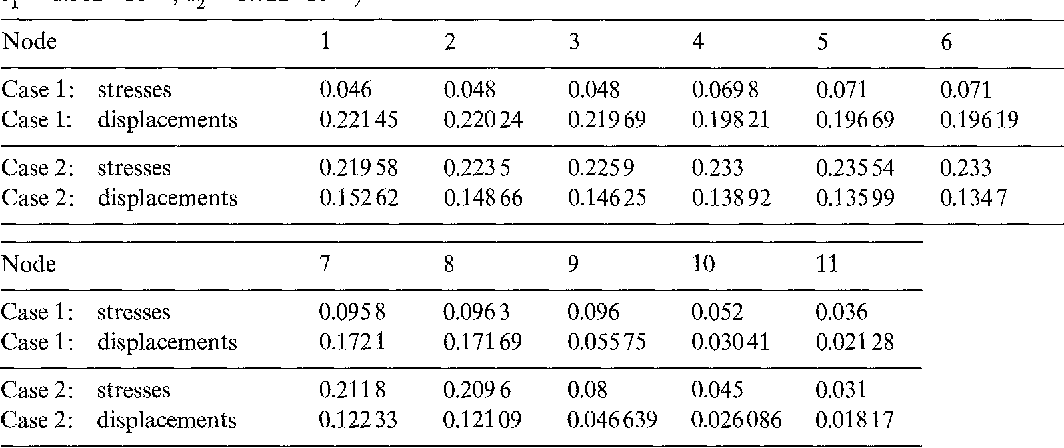Is this relevant?
1989
1989
We investigate delta-convex mappings between normed linear spaces. They provide a generalization of functions which are… Expand
Is this relevant?
1986
1986
This paper considers the problem of minimizing a quasidifferentiable function on a set described by equality-type… Expand
Is this relevant?
1983
1983
The implicit and inverse function theorems of classical differential calculus represent an essential element in the structure of… Expand
Is this relevant?